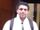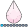Ksharp
Super User

## Fun with SAS ODS Graphics: Tai Chi Graph

It is a special Chinese picture . Have fun.

``````data have;
pi=constant('pi');

/*plot the left white part*/
id=1;
do i=0.5*pi to 1.5*pi by 0.01;
x=cos(i);y=sin(i);output;
end;
do i=1.5*pi to 0.5*pi  by -0.01;
x=0.5*cos(i);y=0.5*sin(i)-0.5;output;
end;
do i=-0.5*pi to 0.5*pi  by 0.01;
x=0.5*cos(i);y=0.5*sin(i)+0.5;output;
end;

/*plot the right dark part*/
id=2;
do i=0.5*pi to -0.5*pi by -0.01;
x=cos(i);y=sin(i);output;
end;
do i=1.5*pi to 0.5*pi  by -0.01;
x=0.5*cos(i);y=0.5*sin(i)-0.5;output;
end;
do i=-0.5*pi to 0.5*pi  by 0.01;
x=0.5*cos(i);y=0.5*sin(i)+0.5;output;
end;

/*plot the  big circle with dark border*/
id=3;
do i=0 to 2*pi by 0.01;
x1=cos(i);y1=sin(i);output;
end;

/*plot the bottom little white pie*/
id=4;
do i=0 to 2*pi  by 0.01;
x2=0.15*cos(i);y2=0.15*sin(i)-0.5;output;
end;

/*plot the top little black pie*/
id=5;
do i=0 to 2*pi  by 0.01;
x3=0.15*cos(i);y3=0.15*sin(i)+0.5;output;
end;
run;

title ;
proc sgplot data=have aspect=1 noautolegend noborder;
styleattrs datacolors=(white black);
polygon x=x y=y id=id/group=id fill   ; /*plot the left and right part*/
polygon x=x2 y=y2 id=id/ fill fillattrs=(color=white)  ; /*plot the bottom little white pie*/
polygon x=x3 y=y3 id=id/ fill fillattrs=(color=black)  ; /*plot the top little black pie*/
series x=x1 y=y1/lineattrs=(color=black thickness=2); /*plot the  big circle with dark border*/
xaxis display=none;
yaxis display=none;
run;``````
` `6 REPLIES 6novinosrin
Tourmaline | Level 20

## Re: Fun with SAS ODS Graphics: Tai Chi GraphKsharp
Super User

## Re: Fun with SAS ODS Graphics: Tai Chi Graphnovinosrin
Tourmaline | Level 20

## Re: Fun with SAS ODS Graphics: Tai Chi Graph

Thank you so much!!!!!!!!!!!djrisks
Barite | Level 11

Nice graph!

## Re: Fun with SAS ODS Graphics: Tai Chi Graph

It is named "Tai Ji" in Chinese

## Re: Fun with SAS ODS Graphics: Tai Chi Graph

Very nice and fun!

Discussion stats
• 6 replies
• 964 views
• 17 likes
• 5 in conversation